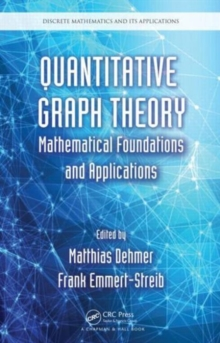Supporting your high street Find out how »
• My AccountQuantitative Graph Theory : Mathematical Foundations and Applications Hardback

Edited by Matthias Dehmer, Frank Emmert-Streib

Description

The first book devoted exclusively to quantitative graph theory, Quantitative Graph Theory: Mathematical Foundations and Applications presents and demonstrates existing and novel methods for analyzing graphs quantitatively.

Incorporating interdisciplinary knowledge from graph theory, information theory, measurement theory, and statistical techniques, this book covers a wide range of quantitative-graph theoretical concepts and methods, including those pertaining to real and random graphs such as:Comparative approaches (graph similarity or distance)Graph measures to characterize graphs quantitativelyApplications of graph measures in social network analysis and other disciplinesMetrical properties of graphs and measuresMathematical properties of quantitative methods or measures in graph theoryNetwork complexity measures and other topological indicesQuantitative approaches to graphs using machine learning (e.g., clustering)Graph measures and statisticsInformation-theoretic methods to analyze graphs quantitatively (e.g., entropy)Through its broad coverage, Quantitative Graph Theory: Mathematical Foundations and Applications fills a gap in the contemporary literature of discrete and applied mathematics, computer science, systems biology, and related disciplines.

It is intended for researchers as well as graduate and advanced undergraduate students in the fields of mathematics, computer science, mathematical chemistry, cheminformatics, physics, bioinformatics, and systems biology.

Information

• Format: Hardback
• Pages: 528 pages, N/A
• Publisher: Taylor & Francis Inc
• Publication Date:
• Category: Combinatorics & graph theory
• ISBN: 9781466584518

£99.99

£87.15

on all orders

Pick up orders

from local bookshops